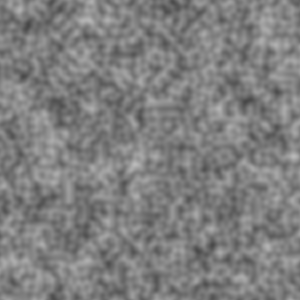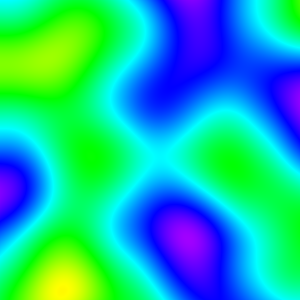# Exploring Perlin Noise in Python

##### Generating coherent noise for all manner of simulations and games

First of all, I would like to say that the code in this post was inspired by Adrian Biagioli’s article on Perlin Noise, which can be found here. That being said, this really isn’t going to be a primer on Perlin Noise itself, rather it’s going to focus on its implementation in Python. First, a recap of the converted C++ code from Adrian’s article:

We start by creating the permutation array which later gives us our ‘random’ gradients. These values are taken directly from Biagioli’s article so we can get similar results, but you can easily randomly generate the gradients (range [0, 255]) for a different approach. We extend `p` by `p` so the indices seem to “wrap around”. Finally we define a `namedtuple` class `Vector`, making xyz-coordinates much easier to handle.

``````from collections import namedtuple

p = [151, 160, 137, 91, 90, 15,
131, 13, 201, 95, 96, 53, 194, 233, 7, 225, 140, 36, 103, 30, 69, 142, 8, 99, 37, 240, 21, 10, 23,
190, 6, 148, 247, 120, 234, 75, 0, 26, 197, 62, 94, 252, 219, 203, 117, 35, 11, 32, 57, 177, 33,
88, 237, 149, 56, 87, 174, 20, 125, 136, 171, 168, 68, 175, 74, 165, 71, 134, 139, 48, 27, 166,
77, 146, 158, 231, 83, 111, 229, 122, 60, 211, 133, 230, 220, 105, 92, 41, 55, 46, 245, 40, 244,
102, 143, 54, 65, 25, 63, 161, 1, 216, 80, 73, 209, 76, 132, 187, 208, 89, 18, 169, 200, 196,
135, 130, 116, 188, 159, 86, 164, 100, 109, 198, 173, 186, 3, 64, 52, 217, 226, 250, 124, 123,
5, 202, 38, 147, 118, 126, 255, 82, 85, 212, 207, 206, 59, 227, 47, 16, 58, 17, 182, 189, 28, 42,
223, 183, 170, 213, 119, 248, 152, 2, 44, 154, 163, 70, 221, 153, 101, 155, 167, 43, 172, 9,
129, 22, 39, 253, 19, 98, 108, 110, 79, 113, 224, 232, 178, 185, 112, 104, 218, 246, 97, 228,
251, 34, 242, 193, 238, 210, 144, 12, 191, 179, 162, 241, 81, 51, 145, 235, 249, 14, 239, 107,
49, 192, 214, 31, 181, 199, 106, 157, 184, 84, 204, 176, 115, 121, 50, 45, 127, 4, 150, 254,
138, 236, 205, 93, 222, 114, 67, 29, 24, 72, 243, 141, 128, 195, 78, 66, 215, 61, 156, 180]
p.extend(p)

Vector = namedtuple('Vector', 'x y z')

``````

We’re now ready to begin implementing the actual Perlin algorithm.

``````import math
def perlin(x, y, z):
arglist = Vector(x, y, z)
if repeat > 0:
arglist = Vector(*map(lambda x: x % repeat, arglist))
float_args, int_args = [Vector(*l) for l in zip(*list(map(math.modf, arglist)))]
int_args = Vector(*map(int, int_args))
...
``````

I kept the function signatures the same as the C++ code (taking 3 `int`s instead of a `Vector`), instead of converting them to take a single `Vector`, so they could be used similarly to how they are in Biagioli’s tutorial. However, once we’ve entered the function, anything is fair game. So we convert them into a `Vector` class, then use `math.modf` to split the values into `int` and `float` parts.

The `fade` function is trivial to implement, as it simply returns 6t5-15t4+10t3, and luckily, we can simply `map` the function over the `float_args` to get all results at once.

``````def fade(t):
return 6*t**5 - 15*t**4 + 10*t**3

def perlin(x, y, z):
...
...

``````

Now, I’ll be the first to admit, my implementation of the `hash` and `inc` functions aren’t very Pythonic, and still carries the C “accent” of the source material. I’m sure there some matrix or combinatoric operation going on here that’s built in. At the very least, I could find a way to rip out the `inc` method and write it straight into the `perlin` function while still maintaining the ‘repeat’ functionality. However, because I understand this part the least, I’m most tempted to leave it alone.

``````def inc(num):
num += 1
if repeat > 0:
num %= repeat
return num

def perlin(x, y, z):
...
aaa = hash_row(*int_args)
aba = hash_row(int_args.x, inc(int_args.y), int_args.z)
aab = hash_row(int_args.x, int_args.y, inc(int_args.z))
abb = hash_row(int_args.x, inc(int_args.y), inc(int_args.z))
baa = hash_row(inc(int_args.x), int_args.y, int_args.z)
bba = hash_row(inc(int_args.x), inc(int_args.y), int_args.z)
bab = hash_row(inc(int_args.x), int_args.y, inc(int_args.z))
bbb = hash_row(*map(inc, int_args))
...
``````

The `grad` function’s goal is to generate the random vectors for the unit cube currently being processed. The article presents two ways of doing this.

``````def grad_slow(hash_list, x, y, z):
h = hash_list & 15
u = x if h < 8 else y
if h < 4:
v = y
elif h == 12 or h == 14:
v = x
else:
v = z
return (u if (h & 1) == 0 else -u) + (v if (h & 2) == 0 else -v)

def grad_fast(hash_list, x, y, z):  # Will be referred to as just 'grad' from now on.
switch = hash_list & 0xF
if switch == 0x0:
return x + y
elif switch == 0x1:
return -x + y
elif switch == 0x2:
return x - y
elif switch == 0x3:
return -x - y
elif switch == 0x4:
return x + z
elif switch == 0x5:
return -x + z
elif switch == 0x6:
return x - z
elif switch == 0x7:
return -x - z
elif switch == 0x8:
return y + z
elif switch == 0x9:
return -y + z
elif switch == 0xA:
return y - z
elif switch == 0xB:
return -y - z
elif switch == 0xC:
return y + x
elif switch == 0xD:
return -y + z
elif switch == 0xE:
return y - x
elif switch == 0xF:
return -y - z
``````

These two functions essentially produce the same results. However, `grad_fast` simply returns the pre-calculated results for the entire state space of `hash_list & 0x0F`. This means we only have to make 1 comparison instead of 6, and one logical AND statement instead of 3. Because of this, henceforth `grad` will refer to `grad_fast`.

Now, we add a quick linear interpolation function, `lerp`.

``````def lerp(a, b, x):
return a + x * (b - a)
``````

And we are ready to put it all together at the end of the `perlin` function.

``````    x1 = lerp(grad(aaa, *float_args), grad(baa, float_args.x - 1, float_args.y, float_args.z), fade_vector.x)
x2 = lerp(grad(aba, float_args.x, float_args.y - 1, float_args.z),
x1 = lerp(grad(aab, float_args.x, float_args.y, float_args.z - 1),
x2 = lerp(grad(abb, float_args.x, float_args.y - 1, float_args.z - 1),

return (lerp(y1, y2, fade_vector.z) + 1) / 2
``````

Interpolating from gradients around the unit cube is what gives it it’s apparent “coherency”.

In order to get a nice output, we try outputting a 2D map image. We should hopefully see some grey blobs

``````import numpy as np
import scipy.misc as smp
# Size of the screen
SCREEN_WIDTH = 300
SCREEN_HEIGHT = 300

# how fine the noise is. lower => finer features
UNIT_CUBE = 128

def main():
starttime = time()
data = np.zeros((SCREEN_WIDTH, SCREEN_HEIGHT, 3), dtype=np.uint8)
# choose a random z-slice to get a random image back. otherwise perlin() always returns the same map (z=0)
z = randint(1, UNIT_CUBE)

for x in range(SCREEN_WIDTH):
for y in range(SCREEN_HEIGHT):
value = perlin(x // UNIT_CUBE + (x % UNIT_CUBE) / UNIT_CUBE, y // UNIT_CUBE + (y % UNIT_CUBE) / UNIT_CUBE,
z)
data[x, y] = list(map(int, (255 * value, 255 * value, 255 * value)))

img = smp.toimage(data)
img.show()
print("Time elapsed: " + str(time() - starttime))
``````

When we’re doing something like `x // UNIT_CUBE + (x % UNIT_CUBE) / UNIT_CUBE` in our call to `perlin`, we’re trying to convert from the pixel coordinate system to the UNIT_CUBE coordinate system. This way, we get nice floating point input numbers for x, y and z.

Your output might look something like this:In order to add more feature diversity to the noise, we can implement the `OctavePerlin` function, which sums together perlin ‘waveforms’ with different `amplitudes` and `frequencies`.

``````def octave_perlin(x, y, z, octaves, persistence):
total = 0
frequency = 1
amplitude = 1
maxValue = 0
for i in range(octaves):
total += perlin(x * frequency, y * frequency, z * frequency) * amplitude
maxValue += amplitude
amplitude += persistence
frequency *= 2
``````

Using the parameters `octaves = 5` and `persistence = 0.75`, I produced this image:This marks the end of the “official” Perlin Tutorial. However, I wasn’t satisfied, and I wanted to add color to the noise (so it would look like a heat-map).

In order to do this, I first need to find a way to map [0.0-1.0] to a color gradient. Luckily, I already had a `HuetoRGB` function around that would convert an angle in degrees [0-360] to an RGB color [0-255][x3]

``````def HueToRGB(h, s=1, v=1):
h = float(h)
s = float(s)
v = float(v)
h60 = h / 60.0
h60f = math.floor(h60)
hi = int(h60f) % 6
f = h60 - h60f
p = v * (1 - s)
q = v * (1 - f * s)
t = v * (1 - (1 - f) * s)
r, g, b = 0, 0, 0
if hi == 0:
r, g, b = v, t, p
elif hi == 1:
r, g, b = q, v, p
elif hi == 2:
r, g, b = p, v, t
elif hi == 3:
r, g, b = p, q, v
elif hi == 4:
r, g, b = t, p, v
elif hi == 5:
r, g, b = v, p, q
r, g, b = int(r * 255), int(g * 255), int(b * 255)
return r, g, b
``````

Then, we add the function to our image generator in `main`:

``````def main():
starttime = time()
data = np.zeros((SCREEN_WIDTH, SCREEN_HEIGHT, 3), dtype=np.uint8)
# choose a random z-slice to get a random image back. otherwise perlin() always returns the same map (z=0)
z = randint(1, UNIT_CUBE)

for x in range(SCREEN_WIDTH):
for y in range(SCREEN_HEIGHT):
value = perlin(x // UNIT_CUBE + (x % UNIT_CUBE) / UNIT_CUBE, y // UNIT_CUBE + (y % UNIT_CUBE) / UNIT_CUBE,
z)  # Currently doesn't work with OctavePerlin
r, g, b = HueToRGB(value * 360)
data[x, y] = list(map(int, (r, g, b)))

img = smp.toimage(data)
img.show()
print("Time elapsed: " + str(time() - starttime))
``````

Running this gives us a beautiful image like this one:The code for this exercise can be found here

In my next Perlin Noise post, I’ll be talking about 3D Noise, and the `noise` python library.

Written on June 8, 2018